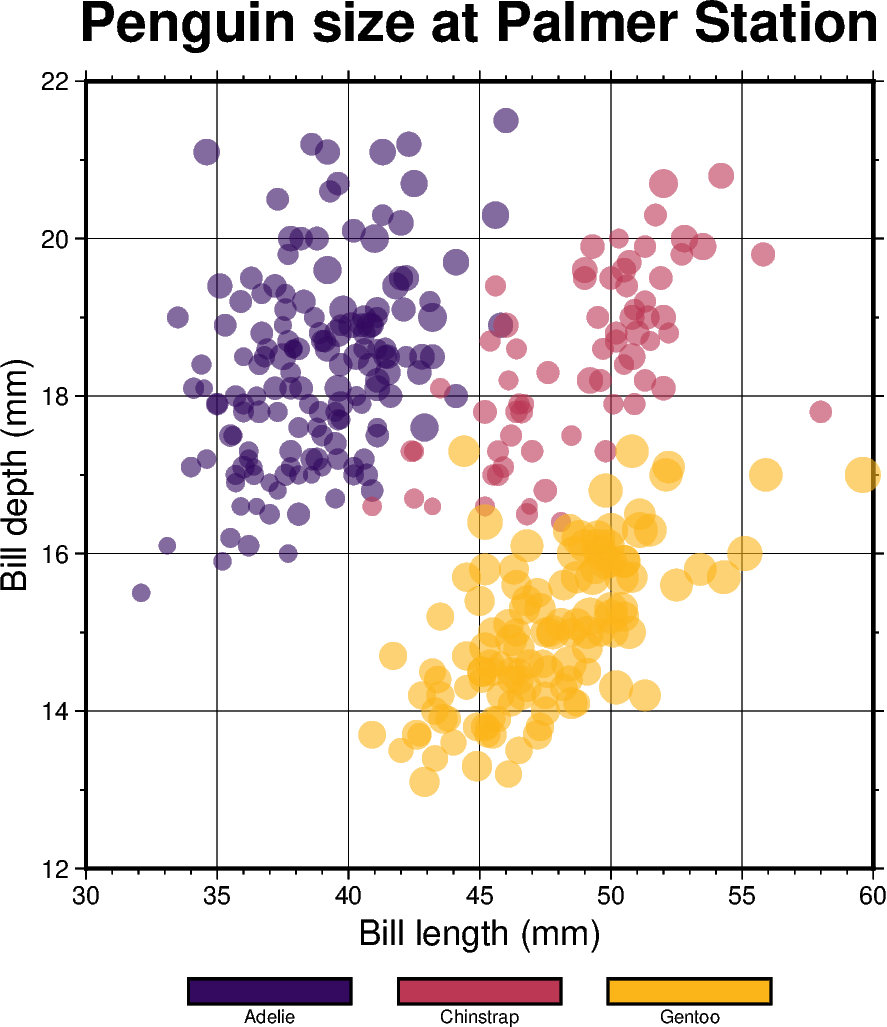# Color points by categories

The `pygmt.Figure.plot` method can be used to plot symbols which are color-coded by categories. In the example below, we show how the Palmer Penguins dataset can be visualized. Here, we can pass the individual categories included in the “species” column directly to the `color` parameter via `color=df.species.cat.codes.astype(int)`. Additionally, we have to set `cmap=True`. A desired colormap can be selected via the `pygmt.makecpt` method.Out:

```<IPython.core.display.Image object>
```

```import pandas as pd
import pygmt

# Convert 'species' column to categorical dtype
# By default, pandas sorts the individual categories in an alphabetical order.
# For a non-alphabetical order, you have to manually adjust the list of
# categories. For handling and manipulating categorical data in pandas,
# have a look at:
# https://pandas.pydata.org/docs/user_guide/categorical.html
df.species = df.species.astype(dtype="category")
# Make a list of the individual categories of the 'species' column
# They are (corresponding to the categorical number code) by default in
# alphabetical order and later used for the colorbar labels
labels = list(df.species.cat.categories)

# Use pygmt.info to get region bounds (xmin, xmax, ymin, ymax)
# The below example will return a numpy array like [30.0, 60.0, 12.0, 22.0]
region = pygmt.info(
data=df[["bill_length_mm", "bill_depth_mm"]],  # x and y columns
per_column=True,  # report the min/max values per column as a numpy array
# round the min/max values of the first two columns to the nearest multiple
# of 3 and 2, respectively
spacing=(3, 2),
)

# Make a 2D categorical scatter plot, coloring each of the 3 species
# differently
fig = pygmt.Figure()

# Generate a basemap of 10 cm x 10 cm size
fig.basemap(
region=region,
projection="X10c/10c",
frame=[
'xafg+l"Bill length (mm)"',
'yafg+l"Bill depth (mm)"',
'WSen+t"Penguin size at Palmer Station"',
],
)

# Define a colormap to be used for three categories, define the range of the
# new discrete CPT using series=(lowest_value, highest_value, interval),
# use color_model="+cAdelie,Chinstrap,Gentoo" to write the discrete color
# palette "inferno" in categorical format and add the species names as
# annotations for the colorbar
pygmt.makecpt(
cmap="inferno",
# Use the minimum and maximum of the categorical number code
# to set the lowest_value and the highest_value of the CPT
series=(df.species.cat.codes.min(), df.species.cat.codes.max(), 1),
color_model="+c" + ",".join(labels),
)

fig.plot(
# Use bill length and bill depth as x and y data input, respectively
x=df.bill_length_mm,
y=df.bill_depth_mm,
# Vary each symbol size according to another feature (body mass,
# scaled by 7.5*10e-5)
size=df.body_mass_g * 7.5e-5,
# Points colored by categorical number code
color=df.species.cat.codes.astype(int),
# Use colormap created by makecpt
cmap=True,
# Do not clip symbols that fall close to the map bounds
no_clip=True,
# Use circles as symbols with size in centimeter units
style="cc",
# Set transparency level for all symbols to deal with overplotting
transparency=40,
)